# Darbo fixed-point theorem

(diff) ← Older revision | Latest revision (diff) | Newer revision → (diff)

The notion of "measure of non-compactness" was first introduced by C. Kuratowski [a1]. For any bounded setin a metric space its measure of non-compactness, denoted by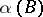, is defined to be the infimum of the positive numberssuch thatcan be covered by a finite number of sets of diameter less than or equal to.

Another measure of non-compactness is the ball measure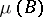, or Hausdorff measure, which is defined as the infimum of the positive numberssuch thatcan be covered by a finite number of balls of radii smaller than. See also Hausdorff measure.

Roughly speaking, a measure of non-compactness is some function defined on the family of all non-empty bounded subsets of a given metric space such that it is equal to zero on the whole family of relatively compact sets.

G. Darbo used a measure of non-compactness to investigate operators whose properties can be characterized as being intermediate between those of contraction and compact mappings (cf. also Compact mapping; Compact operator; Contraction). He was the first to use the indexin the theory of fixed points [a2]. Darbo's fixed-point theorem is a generalization of the well-known Schauder fixed-point theorem (cf. also Schauder theorem). It states that ifis a non-empty bounded closed convex subset of a Banach spaceandis a continuous mapping such that for any set,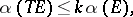(a1)

whereis a constant,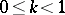, thenhas a fixed point. This theorem is true for the measurealso.

Note that every completely-continuous mapping (or compact mapping; cf. also Completely-continuous operator) satisfies (a1) with, while all Lipschitz mappings with constant(cf. Lipschitz condition) also satisfy (a1). Further, mappings that are not completely continuous but satisfy the condition (a1) are of the form, where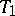is completely continuous and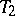satisfies the Lipschitz condition with constant. The significance of this type of mapping is due to the fact that compactness of either the domain or the range is not required.

Methods for determining the value offor a given setin a Banach space are given in [a3].

Darbo's fixed-point theorem is useful in establishing the existence of solutions of various classes of differential equations, especially for implicit differential equations, integral equations and integro-differential equations, see [a3]. It is also used to study the controllability problem for dynamical systems represented by implicit differential equations [a4].

How to Cite This Entry:
Darbo fixed-point theorem. Encyclopedia of Mathematics. URL: http://encyclopediaofmath.org/index.php?title=Darbo_fixed-point_theorem&oldid=19165
This article was adapted from an original article by Krishnan Balachandran (originator), which appeared in Encyclopedia of Mathematics - ISBN 1402006098. See original article